# Sampling and Homology via Bottlenecks

Sampling an algebraic variety and computing its homology

The paper Sampling and homology via bottlenecks by Di Rocco et. al. introduces a novel algorithm for producing a provably dense sample of a smooth and compact algebraic variety. Using the $\textit{bottlenecks}$ we obtain bounds on the density of the sample needed in order to guarantee that the homology of the variety can be recovered from the sample. This example implements the algorithm for the case when the variety is a smooth and compact complete intersection.

## Sampling

By a provably dense sample we mean in the sense of the following definition:

$\textbf{Definition}.$ A $\textit{sample}$ of a variety $X\subset\Bbb R^n$ is a finite subset $E\subset X$. For $\epsilon>0$ a sample $E\subset X$ is called an $\epsilon$-sample if for every $x\in X$ there is an element $e\in E$ such that

$$|x-e|<\epsilon.$$

In this case we also say that $E$ is $\epsilon$-dense.

We will consider the following plane curve, $X$, defined by the following equation: $$(x_1^3 - x_1x_2^2 + x_2 + 1)^2 (x_1^2 + x_2^2 - 1) + x_2^2 - 5=0$$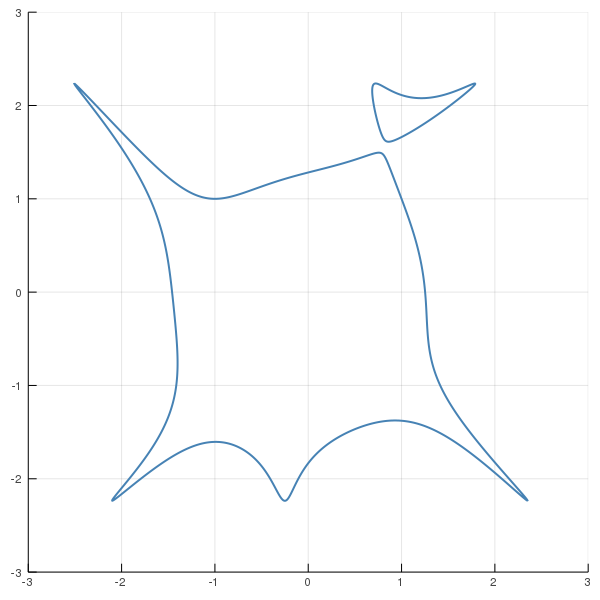First we define the equation of the curve in Julia and list some packages that we will need.
using HomotopyContinuation, DynamicPolynomials, LinearAlgebra, IterTools
n=2 # ambient dimension
@var x[1:n] y[1:n] p[1:n] γ[1:n]

F = [(x^3 - x*x^2 + x + 1)^2 * (x^2 + x^2 - 1) + x^2 - 5]


To produce an $\epsilon$-sample from $X$ we start from its defining equations. The basic idea is then to intersect the variety with a grid of linear spaces of complementary dimension. Figure (A) below show a curve in $\Bbb R^2$ intersected with a 2-dimensional grid.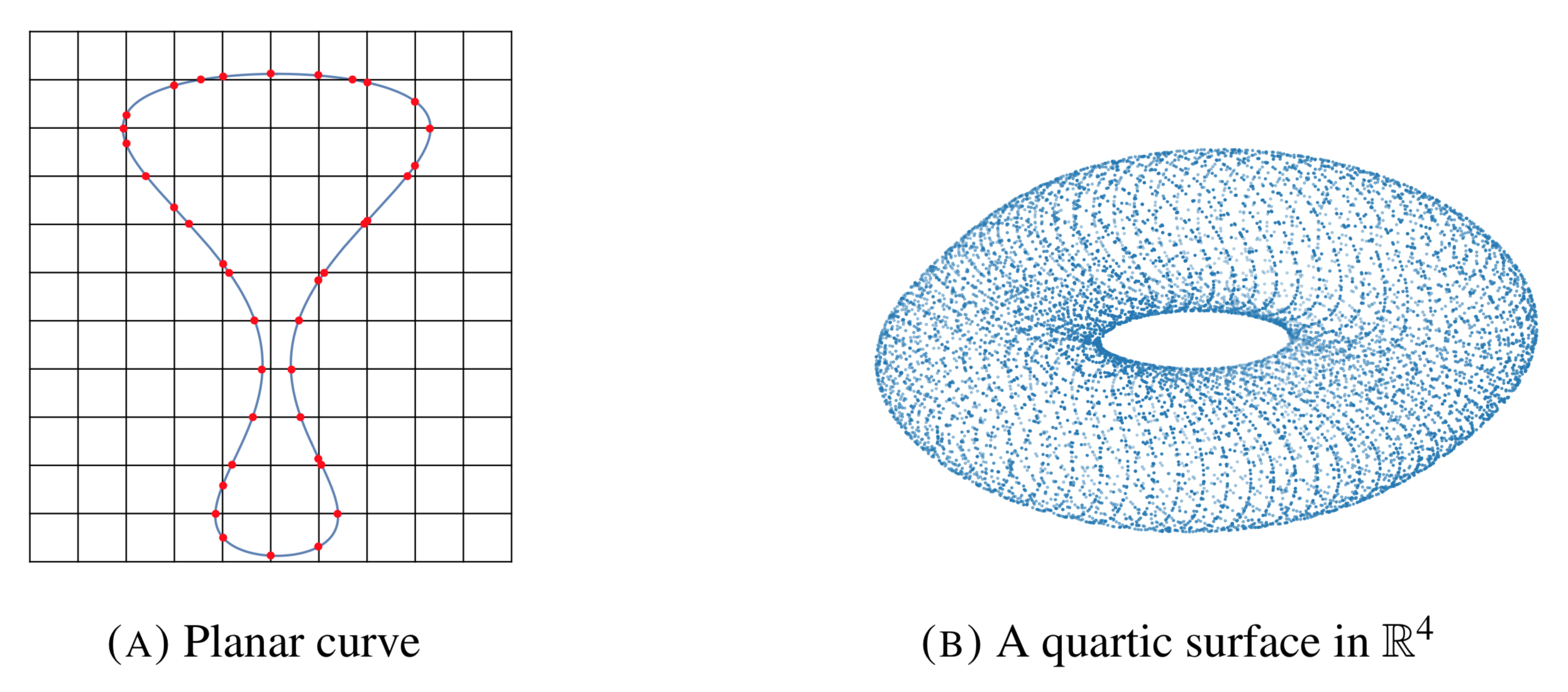However, to obtain a dense sample it is not enough to intersect with linear spaces of complementary dimension. The density guarantee is achieved by introducing extra sample points given by ad hoc slicing and nearest point computations (see Di Rocco et. al. for details). Figure (B) shows an $\epsilon$-sample of a surface in $\Bbb R^4$ with $\epsilon=0.1$.

The grid-size is determined by the density needed, but in order to obtain a dense sample we need the grid-size to be at most the size of the narrowest so called $\textit{bottleneck}$ of the variety.

$\textbf{Theorem}.$ Let $b_2$ be the width of the narrowest bottleneck of $X$ and let $\epsilon > 0$. If the grid size is $\delta$ such that $0<\delta \sqrt{n} < \min(\epsilon, 2b_2)$, then the sampling algorithm returns an $\epsilon$-sample of $X$.

A bottleneck of $X$ is a pair of distinct points $x,y \in X$ such that $(x-y)$ is normal to $X$ at both $x$ and $y$. Next, we compute the bottlenecks of $X$:

# Compute bottlenecks
d=length(F) # codimension of variety
k = n-d # dimension of variety
@var λ[1:d] μ[1:d]; # lagrange multipliers
∇ = differentiate(F, x)
G = subs(F, x => y)
∇y = subs(∇, x => y)
system = [F; G; map(j -> x[j]-y[j]-dot(λ, ∇[:, j]), 1:n); map(j -> x[j]-y[j]-dot(μ, ∇y[:, j]), 1:n)]
result = solve(system)

# pick out the smallest bottleneck
bottlenecks = map(s -> (s[1:n], s[n+1:2*n]), real_solutions(nonsingular(result)))
bn_lengths = sort!(map(b -> (norm(b-b), b), bottlenecks), by = a -> a)
δ = bn_lengths/(2*sqrt(n)) # grid-size


Our next step is to setup the grid that we will use to sample $X$ and for this we need to compute a bounding box that contains $X$. We compute the bounding box by choosing the center of the box, $q$, (which we will choose as the center of the largest bottleneck from the previous computation). We then compute the point on $X$ which is furthest from $q$, the length from $q$ to this point will give us the size of the bounding box.

q = bn_lengths[end] + (bn_lengths[end]-bn_lengths[end])/2
system = [F; map(j -> x[j]-q[j]-dot(λ, ∇[:, j]), 1:n)]
result = solve(system)

critical_points = sort!(map(c -> (norm(c[1:n]-q), c[1:n]), real_solutions(nonsingular(result))), by = a -> a)
b = critical_points[end] # length of bounding box
indices = [i for i in -b:δ:b]


Now we have computed the information we need in order to run the sampling algorithm. The sampling algorithm first intersects $X$ with linear spaces of complementary dimension coming from the grid. It then proceeds to compute the extra sample, which intersects $X$ with linear spaces of higher dimension in order to guarantee that the sample is indeed dense. However, since $X$ has dimension one the extra sample will in this case be empty.

# Basic sample
samples = []
for s in IterTools.subsets(1:n, k)
Ft = [F; map(i -> x[s[i]]-p[i]-q[s[i]], 1:k)]
p₀ = randn(ComplexF64, k)
S_p₀ = solutions(solve(subs(Ft, p[1:k] => p₀)))
params = [[indices[jj] for jj in p1] for p1 in Iterators.product(map(j-> 1:length(indices), s)...)][:]

result = solve(
Ft,
S_p₀;
parameters = p[1:k],
start_parameters = p₀,
target_parameters = params,
transform_result = (_r, _p) -> real_solutions(_r),
flatten = true
)
samples = vcat(samples, result)
end

# Extra sample
for l in 1:k-1
for s in IterTools.subsets(1:n, l)
Ft = [F; map(i -> x[s[i]]-p[i]-q[s[i]], 1:l)]
∇ = differentiate(Ft, x)
Ft = [Ft; map(j -> x[j]-y[j]-dot(γ[1:n-k+l], ∇[:, j]), 1:n)]

p₀ = randn(ComplexF64, n+l)
S_p₀ = solutions(solve(subs(Ft, [y; p[1:l]] => p₀)))
params = [vcat(randn(Float64, n), [indices[jj] for jj in p1]) for p1 in Iterators.product(map(j-> 1:length(indices), s)...)][:]

result = solve(
Ft,
S_p₀;
parameters = [y; p[1:l]],
start_parameters = p₀,
target_parameters = params,
transform_result = (_r, _p) -> real_solutions(_r),
flatten = true
)
samples = vcat(samples, result)
end
end


The result of the sampling algorithm is shown below:

using Plots
sample_matrix = hcat(samples...)
scatter(sample_matrix[1,:], sample_matrix[2,:],
legend = false)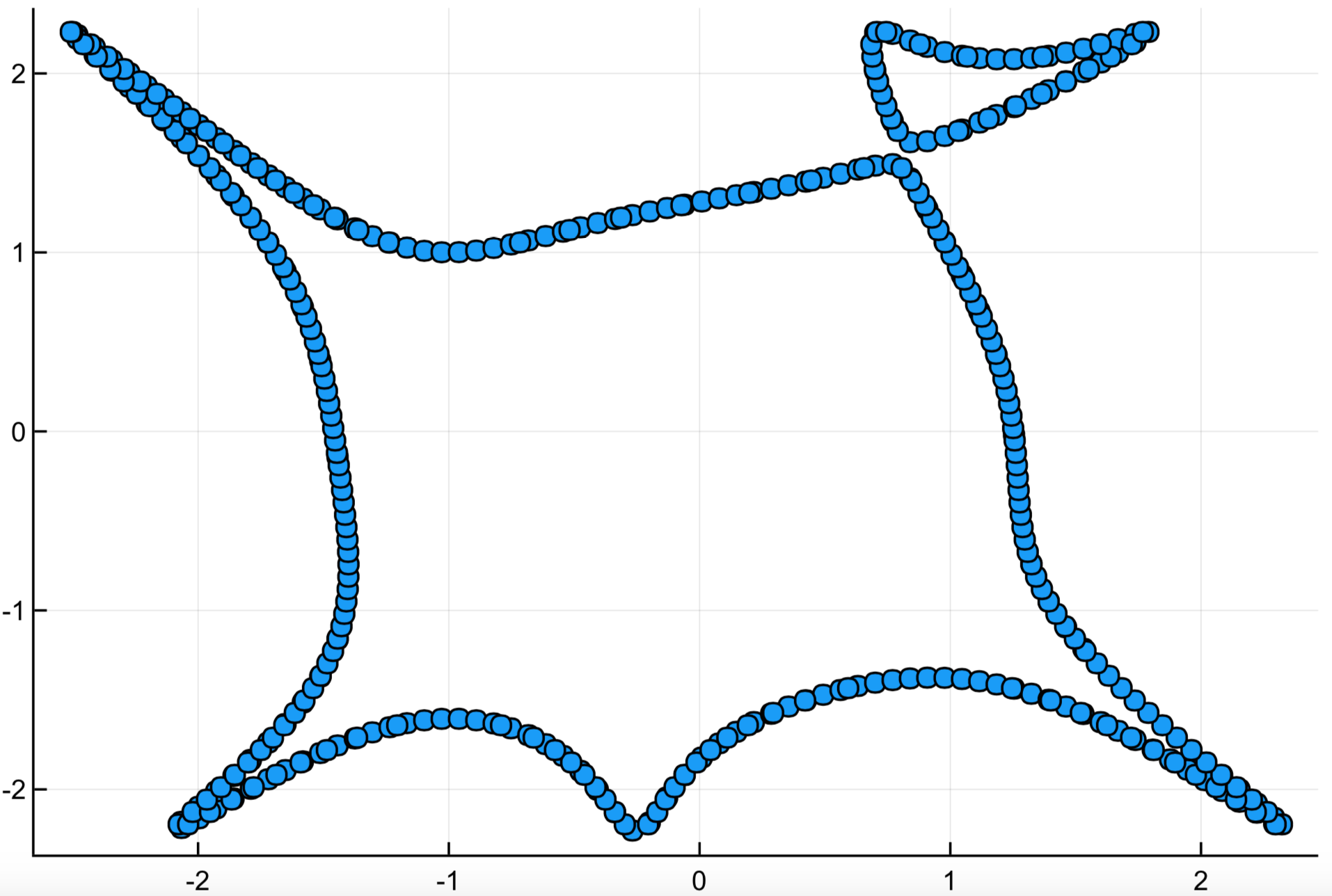## Homology

Using the sample we may now recover the homology of $X$. It is shown by Di Rocco et. al. that in order to recover the zeroth and first homology of $X$ it is sufficient with a density less than the width of the narrowest bottleneck of $X$. This follows from the following theorem and from the fact that the narrowest bottleneck of $X$ equals the $\textit{weak feature size (wfs)}$ in this case (see Di Rocco et. al. for details).

$\textbf{Theorem}.$ Let $\epsilon < wfs(X)$ and let $i\in \{0, 1\}$. Let $E$ be an $\epsilon$-dense sample of $X$ and let $S$ be the modified Vietoris-Rips complex constructed from $E$ below. Then, $$H_i(S) \cong H_i(X)$$

Recovering the homology of from such an $\epsilon$-sample of $X$ requires us to construct a special modification of a Vietoris-Rips complex, which is shown to have the same zeroth and first homology as $X$. We next proceed to compute this modified Vietoris-Rips complex and compute its homology using the software package Eirene. First we compute the distance matrix of the sample:

using Eirene
ϵ = bn_lengths #Two times the width of the narrowest bottleneck
D = zeros((length(samples), length(samples))) #Initialize distance matrix

neighbour_lists = []
for i in 1:length(samples)
push!(neighbour_lists, [])
end
candidate_lists = []

for i in 1:length(samples)
candidate_list = []
for j in (i+1):length(samples)
dist = norm(samples[i]-samples[j])
if dist < sqrt(8)*ϵ
if dist < ϵ
push!(neighbour_lists[i], j)
push!(neighbour_lists[j], i)
else
push!(candidate_list, j)
end
end
D[i, j] = dist
D[j, i] = dist
end
push!(candidate_lists, candidate_list)
end



The computation of the modified Vietoris-Rips complex can be implemented as a preprocessing step on the distance matrix. To compute the modified Vietoris-Rips complex one then computes the Vieoris-Rips complex from the modified distance matrix. Next we perform this modification of the distance matrix:

DM = deepcopy(D) #Modified distance matrix
thresh = ϵ - 10^(-10)
for i in 1:length(samples)
for j in candidate_lists[i]
for k in neighbour_lists[j]
if D[i, k] < ϵ && D[j, k] < ϵ
DM[i, j] = thresh
DM[j, i] = thresh
break
end
end
end
end


Finally we compute the homology of the complex using Eirene.

H = eirene(DM, model="vr", maxdim=1, minrad=ϵ, maxrad=ϵ);


To verify the result we extract the Betti-numbers:

using PrettyTables
pretty_table([ betticurve(H, dim=0)[1, 2] betticurve(H, dim=1)[1, 2]], ["β_0" "β_1"])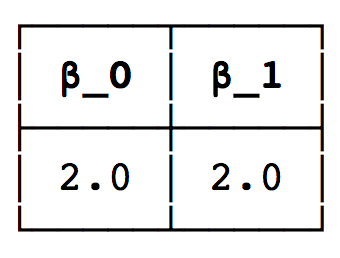To compute the higher homology groups for varieties of larger dimension we make use of local reach estimations. Let $U(E, \epsilon)$ denote the union of all $\epsilon$-balls $B_\epsilon(e)$ for $e\in E$.

$\textbf{Theorem}.$ If $E$ is a $(\epsilon/2)$-sample of $X$ and $\epsilon < \frac{4}{5}\tau_X(e)$ for all $e \in E$, then $X$ and the $\textit{\u{C}ech complex}$ of $U(E, \epsilon)$ have the same homology groups.

The local reach $\tau_X(e)$ can be bounded from below using a result from Schub Smale theory (see Di Rocco et. al. Section 3.3) which says that $$\frac{1}{7D^{3/2}\mu_{\text{norm}}(F,e)} \leq \tau_X(e)$$ where $F$ is the system of equations defining $X$, $D$ is the maximum degree of the defining equations and $\mu_{\text{norm}}$ is the condition number of $F$ at $e$. Define

$$\eta(e)^{-1} := 7D^{3/2}\mu_{\text{norm}}(F,e).$$

To find a sampling density $\epsilon$ which satisfies the requirements of the above theorem we formulate the following algorithm: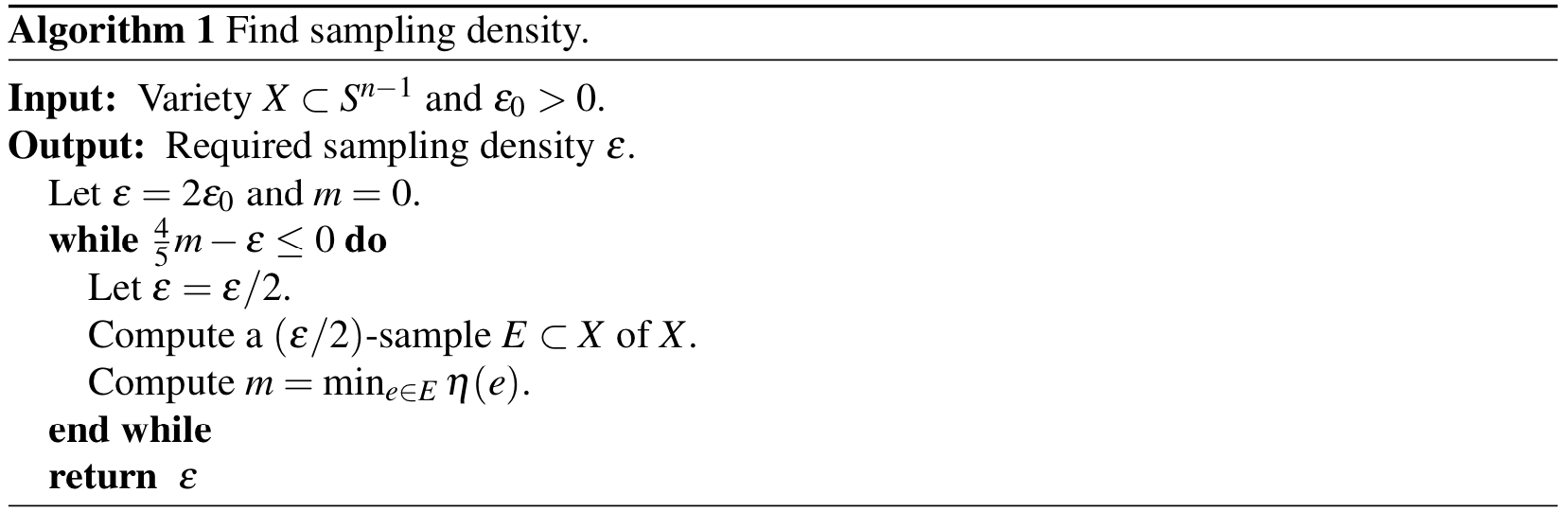Cite this example:
@Misc{ sampling_bottlenecks2022 ,
author =  { Oliver Gäfvert },
title = { Sampling and Homology via Bottlenecks },
howpublished = { \url{ https://www.JuliaHomotopyContinuation.org/examples/sampling_bottlenecks/ } },
note = { Accessed: June 27, 2022 }
}